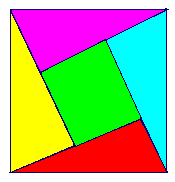# Problem 100 AnswerGiven any right triangle you can create a figure like the one above using four of them. This is because the sum of the three angles always is 180 degrees. The area of the outside square is c2. We can also find the area by summing the area of the individual parts: 4*(a*b/2)+(a-b)2 = 2ab + a2 -2ab + b2 = a2 + b2. Thus a2 + b2 = c2.

I didn't assign a diffulty level because I saw Carl Sagan explain this proof on television so I never had a chance to solve it myself.

Michael Shackleford, A.S.A.